Categories

# Acute angles between surfaces: IIT JAM 2018 Qn 6

This is an application analysis question that appeared in IIT JAM 2018. The concept required is the multivarible calculus and vector analysis.

[h5p id="6"]

# Understand the problem

In$\Bbb R^3$ the cosine of acute angle between the surfaces$x^2+y^2+z^2-9=0$ and$z-x^2-y^2+3=0$ at the point$(2,1,2)$ is
1.$\frac{8}{5\sqrt{21}}$
2.$\frac{10}{5\sqrt{21}}$
3.$\frac{8}{3\sqrt{21}}$
4.$\frac{10}{3\sqrt{21}}$
##### Source of the problem
IIT JAM 2018 Qn no 6
##### Topic
Multivable calculus
Easy
##### Suggested Book
 Calculus: Multi-Variable Calculus and Linear Algebra with Applications to Differential Equations and Probability – Vol 2 Tom M. Apostol

Do you really need a hint? Try it first!

If we are asked to give the angle between two lines then it is very easy to calculate but our forehead will get skinned whenever we will be asked to find out the acute angle between two lines and even worse if we are asked to find the angle between two surfaces.   Surprisingly it is not very hard if think stepwise. Observe when you are asked to find out the angle between two lines you calculate it in terms slope. So basically you are firing putting the gun on someone else’s shoulder. Here the question is to find that shoulder when it comes in finding the angle between two curves. Observe from the conception of the intersection of two curves that the tangent line of those curves also intersects and we have their corresponding slopes. Bingo! why not calculating the acute angle of the tangent lines and call them the angle between two curves.   Now can you think how to calculate the acute angle between to surfaces?
The acute angle between two surfaces would be the acute angle between their tangent plane. You can stop here and try to do the problem by your own otherwise continue…   The main idea of finding tangent planes revolves around finding gradient of the corresponding surfaces. (For more info see question no 5).   Can you calculate the gradient of the surfaces$x^2+y^2+z^2-9$ and$z-x^2-y^2+3$ at$(2,1,2)$?
The gradient of the surfaces$f=x^2+y^2+z^2-9$ and$g=z-x^2-y^2+3$ at$(2,1,2)$ are$n_1=f_xi +f_yj+f_zk$ and$n_2=g_xi +g_yj+g_zk$ at$(2,1,2)$ which is$n_1=2xi+2yj+2zk=4i+2j+4k$  and$n_2=-2xi-2yj+k=-4i-2j+k$.   Now given these two gradients, can you find out the angle between them?$n_1=2xi+2yj+2zk=4i+2j+4k$  and$n_2=-2xi-2yj+k=-4i-2j+k$.

This follows the cosine angles between two gradient is$cos \theta=|\frac{n_1.n_2}{|n_1||n_2|}|=|\frac{-16-4+4}{\sqrt{36 \times 21}}|=\frac{8}{3\sqrt{21}}$

# Explanation of hints with graph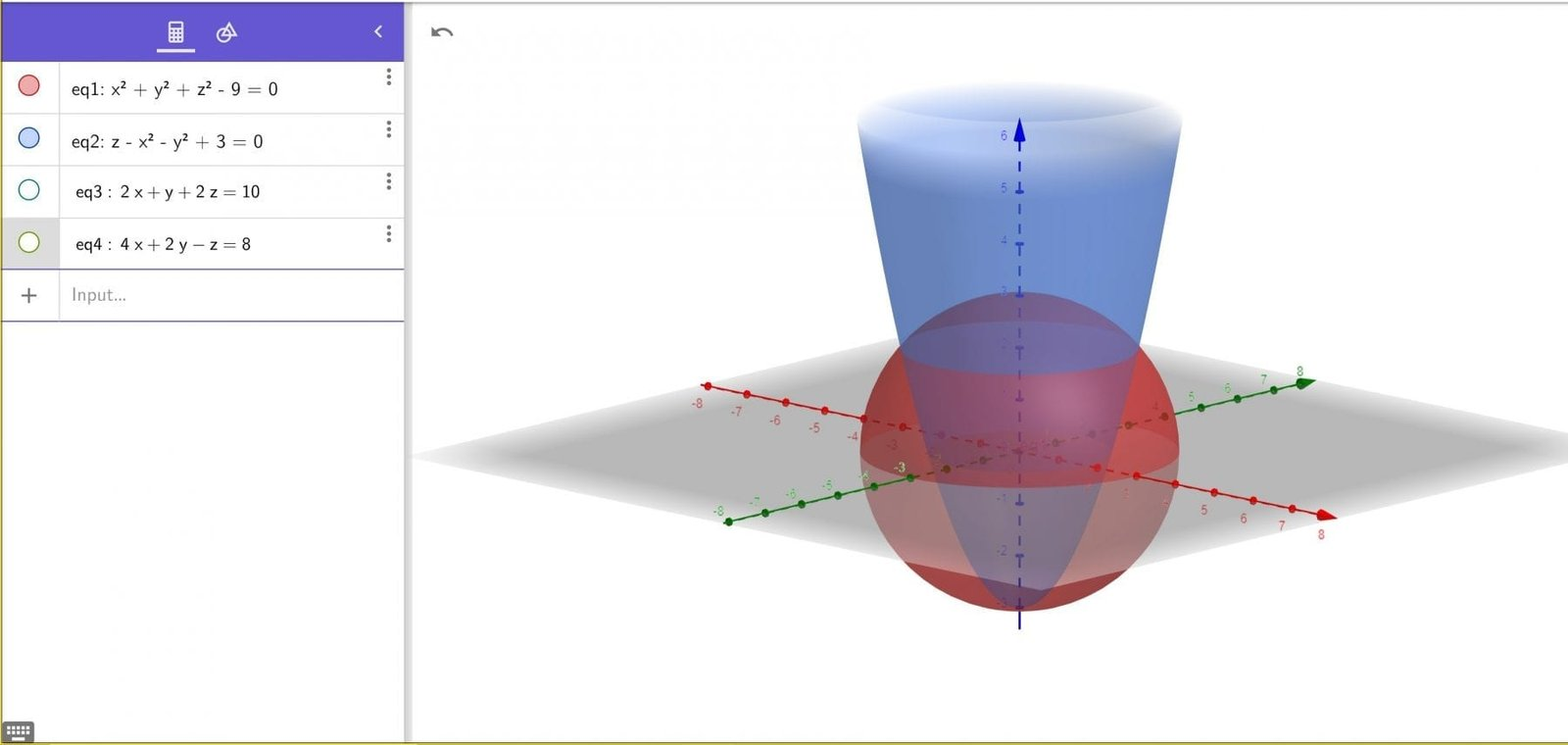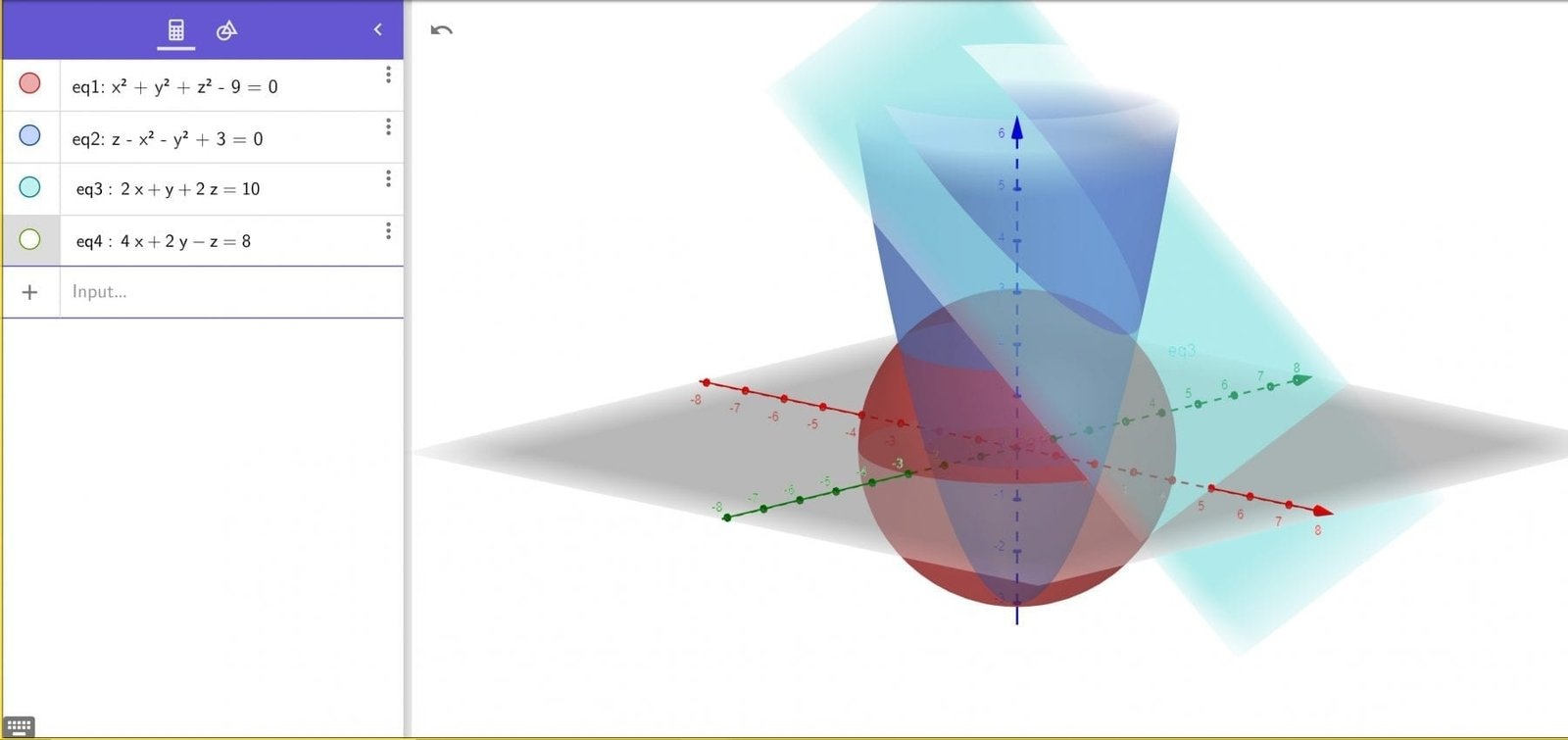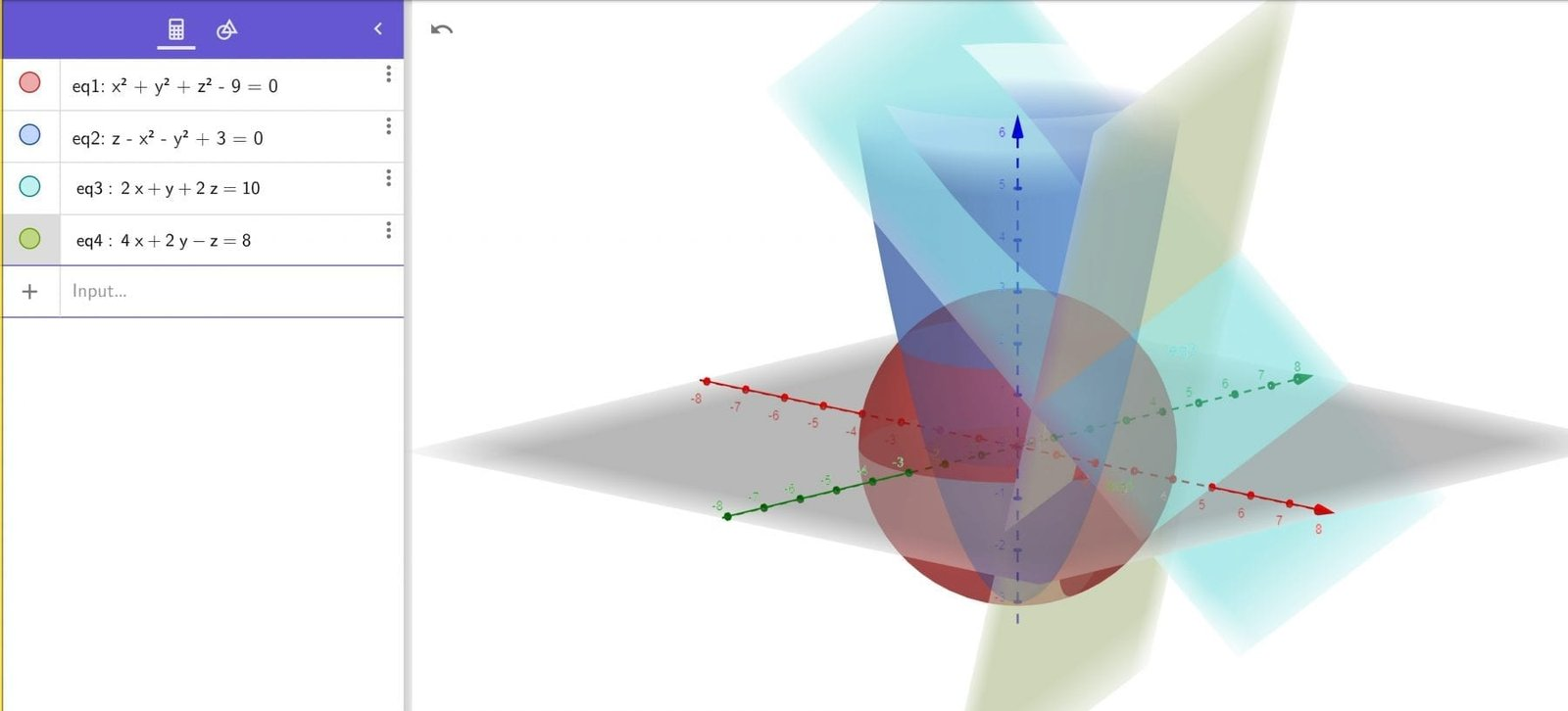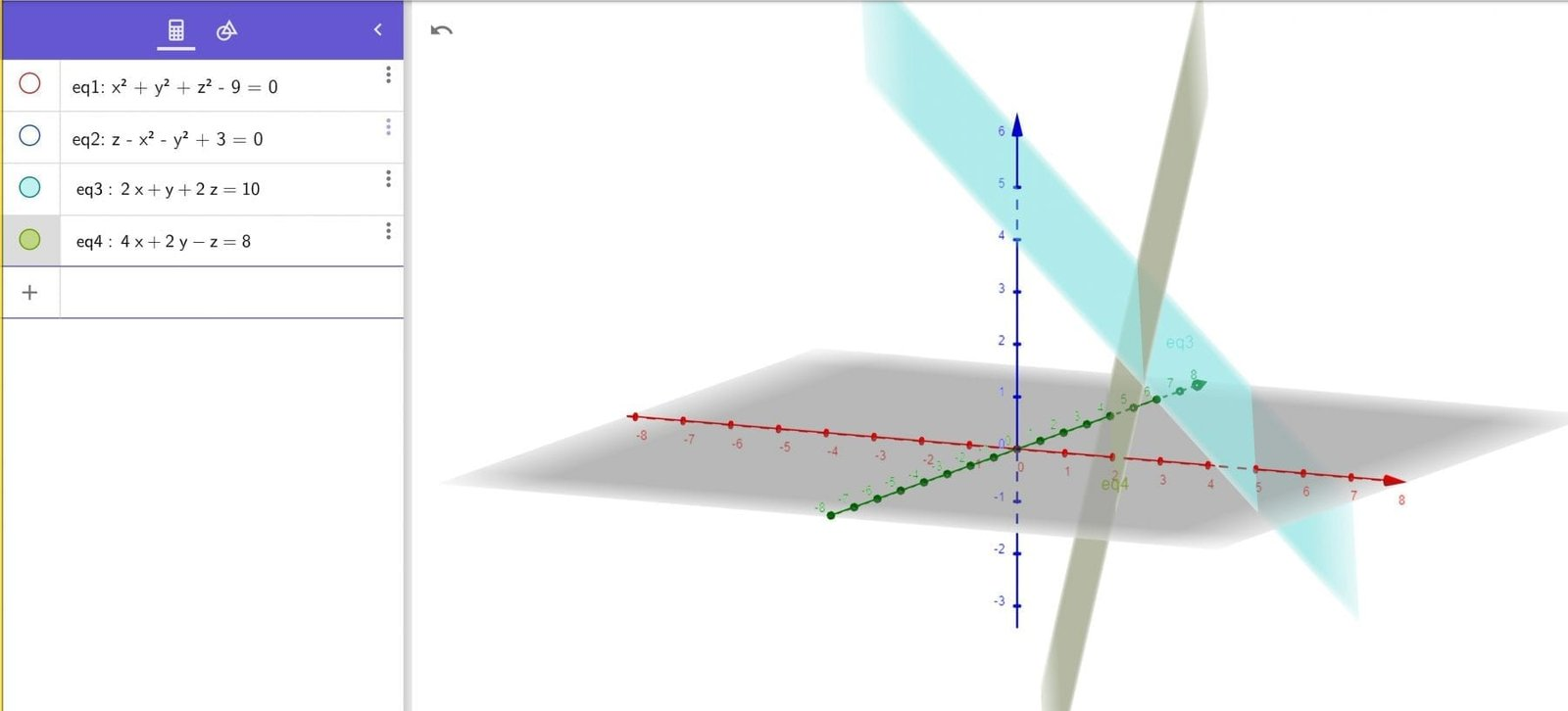# Knowledge Graph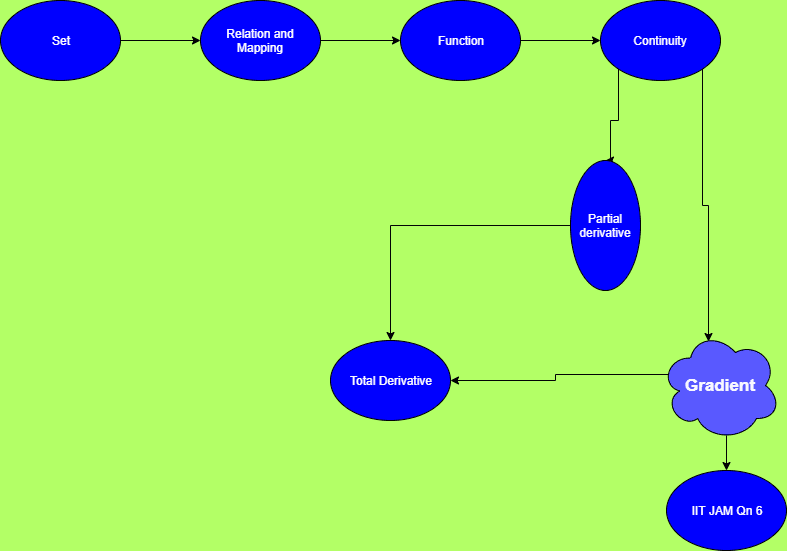# Connected Program at Cheenta

#### College Mathematics Program

The higher mathematics program caters to advanced college and university students. It is useful for I.S.I. M.Math Entrance, GRE Math Subject Test, TIFR Ph.D. Entrance, I.I.T. JAM. The program is problem driven. We work with candidates who have a deep love for mathematics. This program is also useful for adults continuing who wish to rediscover the world of mathematics.

# Similar Problems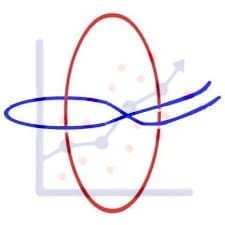## ISI MStat PSB 2006 Problem 8 | Bernoullian Beauty

This is a very simple and regular sample problem from ISI MStat PSB 2009 Problem 8. It It is based on testing the nature of the mean of Exponential distribution. Give it a Try it !## ISI MStat PSB 2009 Problem 8 | How big is the Mean?

This is a very simple and regular sample problem from ISI MStat PSB 2009 Problem 8. It It is based on testing the nature of the mean of Exponential distribution. Give it a Try it !## ISI MStat PSB 2009 Problem 4 | Polarized to Normal

This is a very beautiful sample problem from ISI MStat PSB 2009 Problem 4. It is based on the idea of Polar Transformations, but need a good deal of observation o realize that. Give it a Try it !## ISI MStat PSB 2009 Problem 6 | abNormal MLE of Normal

This is a very beautiful sample problem from ISI MStat PSB 2009 Problem 6. It is based on the idea of Restricted Maximum Likelihood Estimators, and Mean Squared Errors. Give it a Try it !## ISI MStat PSB 2009 Problem 3 | Gamma is not abNormal

This is a very simple but beautiful sample problem from ISI MStat PSB 2009 Problem 3. It is based on recognizing density function and then using CLT. Try it !## ISI MStat PSB 2009 Problem 1 | Nilpotent Matrices

This is a very simple sample problem from ISI MStat PSB 2009 Problem 1. It is based on basic properties of Nilpotent Matrices and Skew-symmetric Matrices. Try it !## ISI MStat PSB 2006 Problem 2 | Cauchy & Schwarz come to rescue

This is a very subtle sample problem from ISI MStat PSB 2006 Problem 2. After seeing this problem, one may think of using Lagrange Multipliers, but one can just find easier and beautiful way, if one is really keen to find one. Can you!

## Problem on Inequality | ISI – MSQMS – B, 2018 | Problem 2a

Try this problem from ISI MSQMS 2018 which involves the concept of Inequality. You can use the sequential hints provided to solve the problem.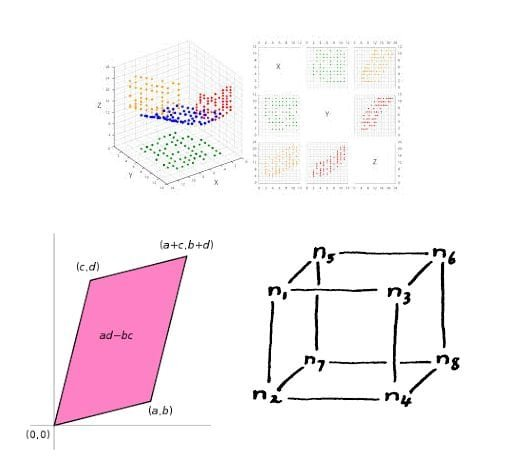## Data, Determinant and Simplex

This problem is a beautiful problem connecting linear algebra, geometry and data. Go ahead and dwelve into the glorious connection.

## Problem on Integral Inequality | ISI – MSQMS – B, 2015

Try this problem from ISI MSQMS 2015 which involves the concept of Integral Inequality and real analysis. You can use the sequential hints provided to solve the problem.

This site uses Akismet to reduce spam. Learn how your comment data is processed.